In pics: Here is the complete list of Oscar winners 2018

First Published 5, Mar 2018, 11:43 AM ISTIn pics: Here is the complete list of Oscar winners 2018
In pics: Here is the complete list of Oscar winners 2018
In pics: Here is the complete list of Oscar winners 2018In pics: Here is the complete list of Oscar winners 2018
In pics: Here is the complete list of Oscar winners 2018In pics: Here is the complete list of Oscar winners 2018
In pics: Here is the complete list of Oscar winners 2018
In pics: Here is the complete list of Oscar winners 2018
In pics: Here is the complete list of Oscar winners 2018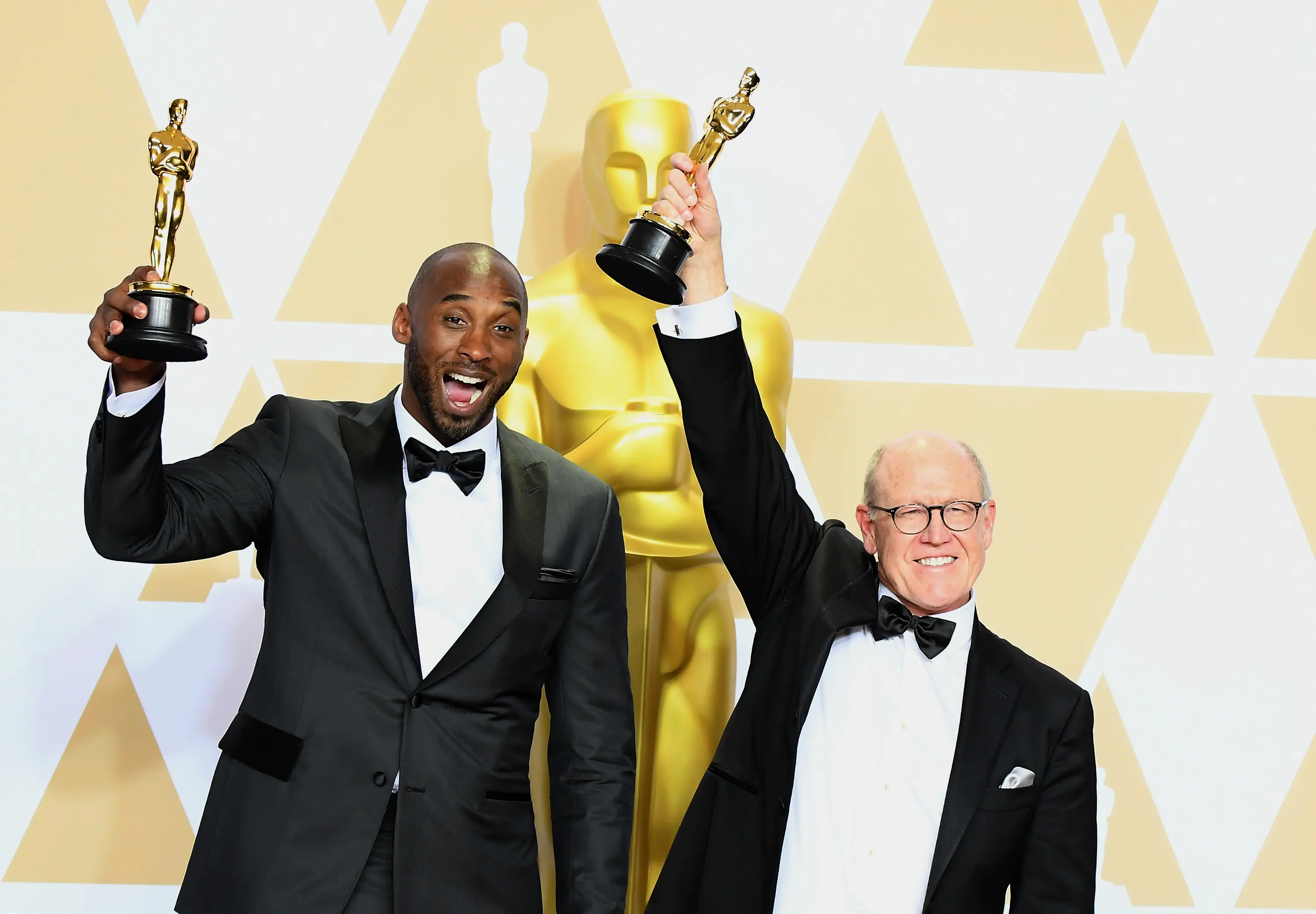In pics: Here is the complete list of Oscar winners 2018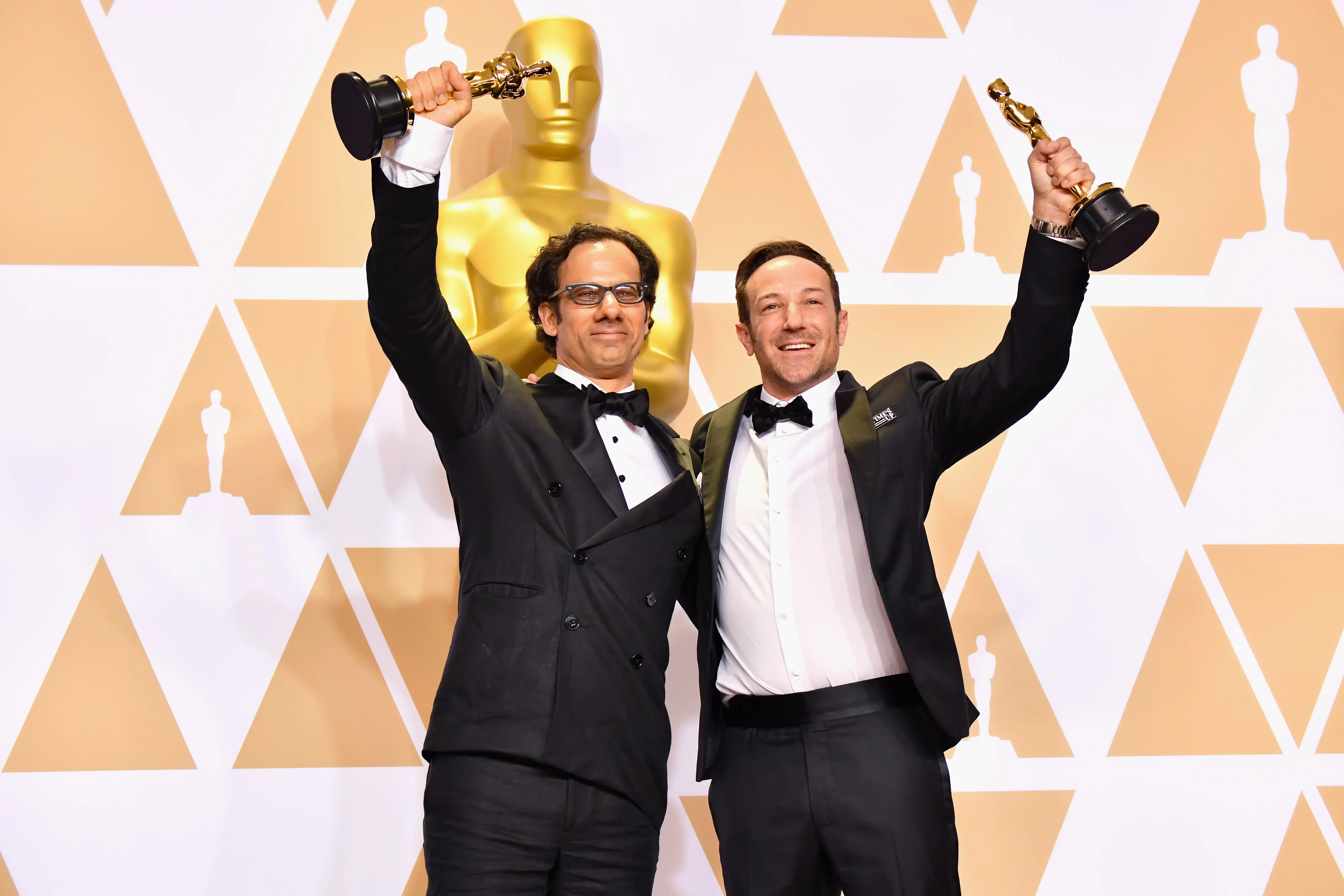In pics: Here is the complete list of Oscar winners 2018
In pics: Here is the complete list of Oscar winners 2018
In pics: Here is the complete list of Oscar winners 2018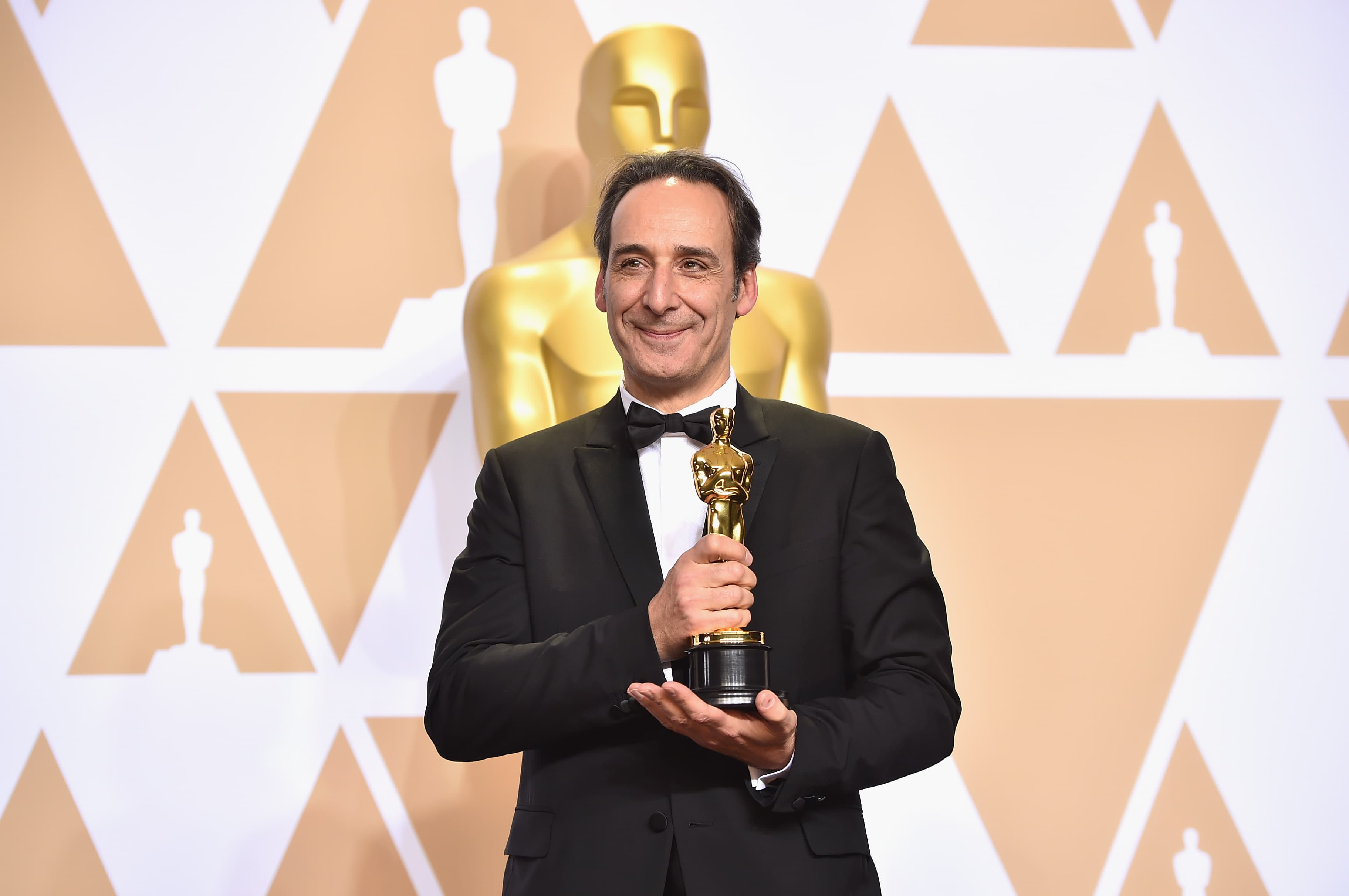In pics: Here is the complete list of Oscar winners 2018
In pics: Here is the complete list of Oscar winners 2018
In pics: Here is the complete list of Oscar winners 2018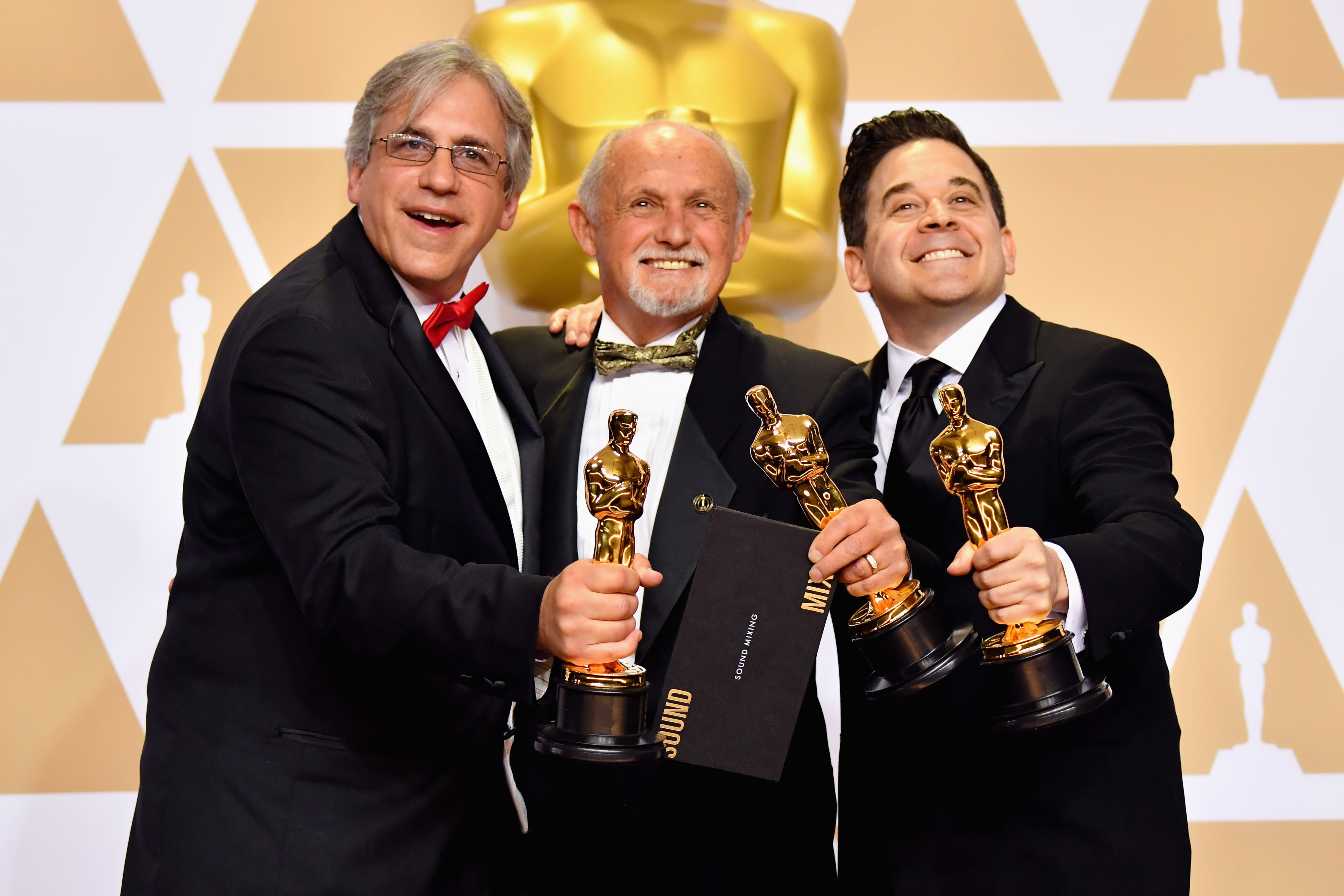In pics: Here is the complete list of Oscar winners 2018
In pics: Here is the complete list of Oscar winners 2018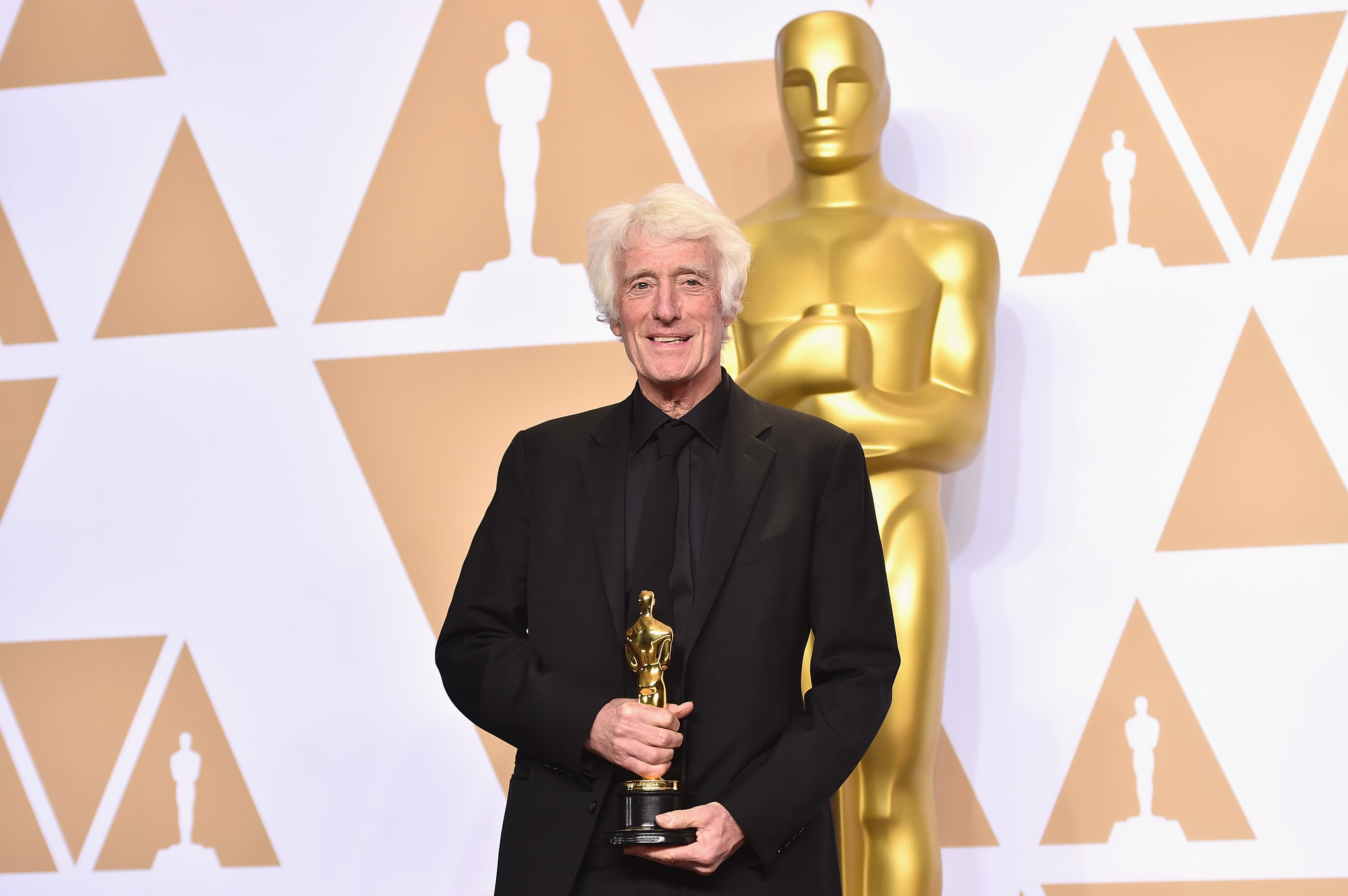In pics: Here is the complete list of Oscar winners 2018
In pics: Here is the complete list of Oscar winners 2018
In pics: Here is the complete list of Oscar winners 2018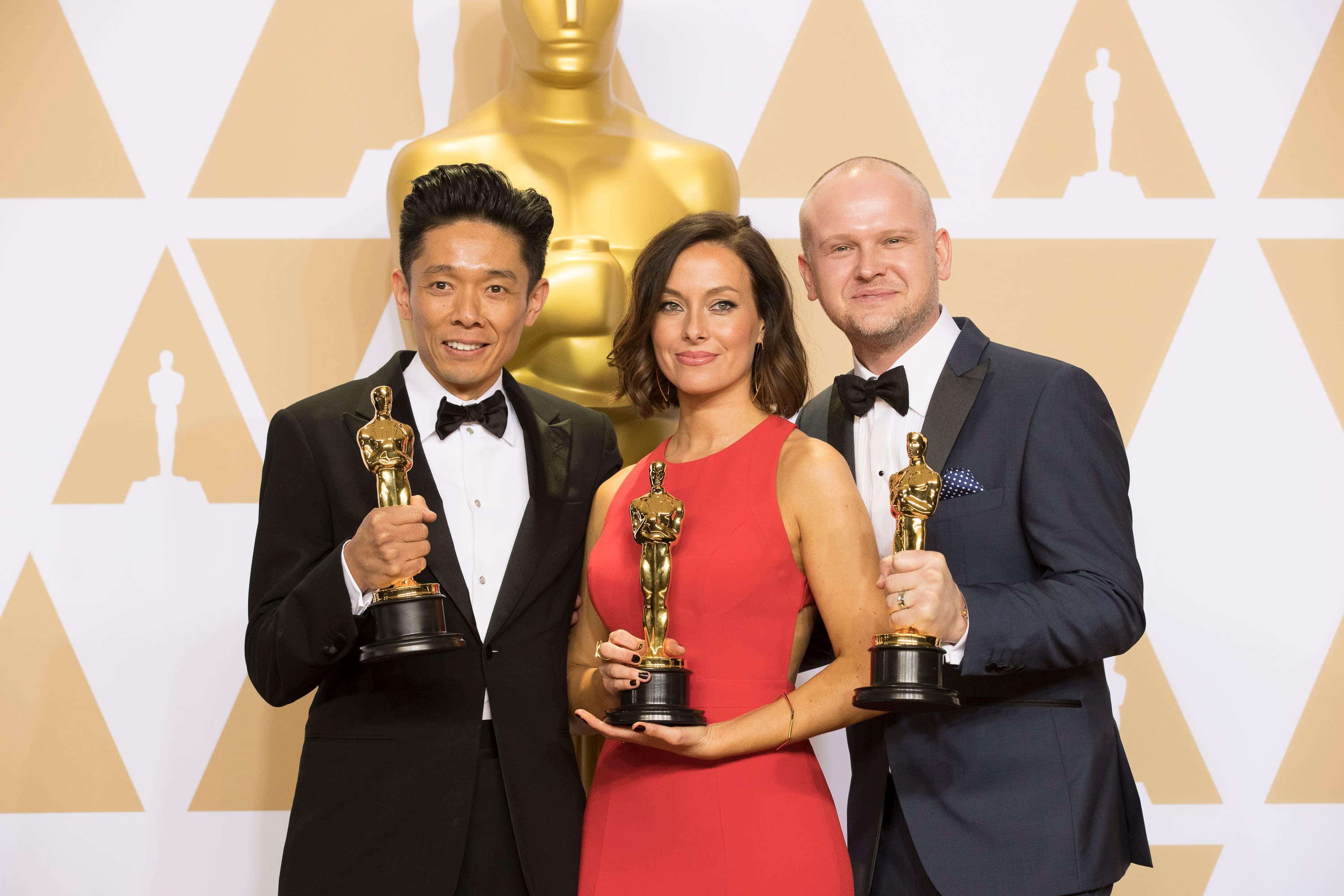In pics: Here is the complete list of Oscar winners 2018